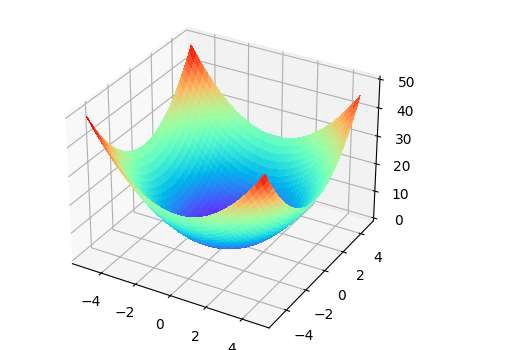# How to display a 3D plot of a 3D array isosurface in matplotlib mplot3D or similar?

Let's take an example to see how to display a 3D plot of a 3D array isosurface in matplotlib −

## Example

import numpy as np
import matplotlib.pyplot as plt
from mpl_toolkits.mplot3d import Axes3D
plt.rcParams["figure.figsize"] = [7.50, 3.50]
plt.rcParams["figure.autolayout"] = True
x = np.arange(-5, 5, 0.25)
y = np.arange(-5, 5, 0.25)
x, y = np.meshgrid(x, y)
h = x ** 2 + y ** 2
fig = plt.figure()
ax = Axes3D(fig)
ax.plot_surface(x, y, h, rstride=1, cstride=1, cmap=plt.cm.rainbow, linewidth=0, antialiased=False)
plt.show()

## Output